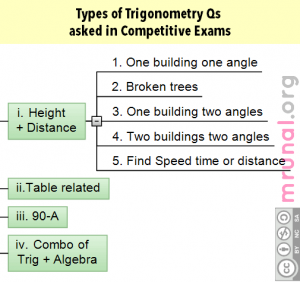# [Trigonometry] Introduction, Types of Questions, Shortcut for memorizing 30,45,60 for SSC CGL CDS

In Aptitude by Support Staff

# Introduction

• From outside, trigonometry may look lengthy, boring, difficult, tedious and frustrating. But it doesn’t change the fact that trigonometry as a topic important for SSC exams (CGL, FCI, CPIO etc.) and CDS exam (Combined defense services).
• In CGL-2012, at both Tier I and II, there were around 8 questions from Trigonometry alone.
• Based on the previous SSC papers, I classify the Trigonometry questions mainly into 4 types

# Types of Trigonometry Questions1. Height and distance related Example “String of a kite is 100 metres long and it makes an angle of 60° with the ground. Find the height at which the kite is flying.” Height and distance questions can be further classified into five subtypes, but most of them can be solved pretty easily via “Tan 30, 45 and 60.” The techniques and shortcuts are explained my youtube videos. 2. Table related For example find value of sin430+cos345*cosec260+…something like that. To solve such questions, you’ve to know the sin cos tan cosec sec and cot values of 0, 30, 45, 60 and 90 angles. Again nothing difficult, as long as you know the values. 3. Complementary angles (90-A) related for example find value of tan4 x tan 43 x tan 47 x tan 86. 4. Combo of Trigonometry formulas + Algebra formulas. For example find value of (1+cotA-cosecA)(1+tanA+secA)

# Trig: Height and Distance

These questions can be further classified into five types

## Example

1. One building one angle From a ground point that is 400 meters away from a building, the angle of elevation is 30 degrees. Find height of this building.
2. Broken trees and telephone poles A tree breaks down due to storm and its top touches the ground and makes an angle of 30°. If the top of that tree touches the ground 15 m away from the bottom then find the height of this tree
3. One building two angles or two shadows. As angle of elevation of the sun increases from 30° to 60°, Shadow of a building is reduced by 10 m find the height of this building.
4. Two buildings two angles From a 60 m tall building, the angle of depression of the top and the bottom of a tower is 30 and 60° respectively. Find the height of this tower.
5. Find Speed time or distance. A man on the top of building observes a car moving at a uniform speed and coming directly towards the building.  It takes 12 minutes for the angle of depression to change from 30 to 45, how soon after this, will the car reach the building?

In the books, you may have seen a big table containing values of sin cos tan cosec sec and cot for 0, 30, 45, 60 and 90 angles. As far as Height and distance is concerned, there is absolutely no need to mugup that big n boring table because of following reasons

1. Most of the questions only have 30, 45 and 60 degrees.
2. And To memorize the values of SIN, COS, TAN, COSEC, SEC and COT for 30, 45, 60, Just remember the Topi TrianglesTM technique. (Explained in following video)

If the video is not visible, check it directly on my youtube channel youtube.com/user/TheMrunalPatel
Above video also contains overview of the Height and Distance Questions and its five subtypes. How to solve each type of height and distance questions, will be explained in depth in separate videos later on. For more [Aptitude] related content, visit the Archive on Mrunal.org/aptitude